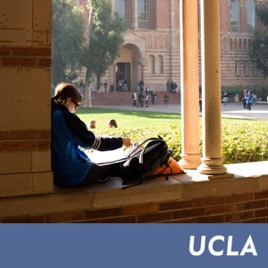27 Folgen

A math course for life science majors covering elementary probability, probability distributions, random variables, and limit theorems.# Mathematics 3C - Video Lectures UCLA

• Bildung

A math course for life science majors covering elementary probability, probability distributions, random variables, and limit theorems.

• video
Probability for Math Science: UCLA Math 3C, Lecture 1

## Probability for Math Science: UCLA Math 3C, Lecture 1

• 44 Min.
• video
Probability for Math Science: UCLA Math 3C, Lecture 2

## Probability for Math Science: UCLA Math 3C, Lecture 2

• 48 Min.
• video
Probability for Math Science: UCLA Math 3C, Lecture 3

## Probability for Math Science: UCLA Math 3C, Lecture 3

• 51 Min.
• video
Probability for Math Science: UCLA Math 3C, Lecture 4

## Probability for Math Science: UCLA Math 3C, Lecture 4

• 49 Min.
• video
Probability for Math Science: UCLA Math 3C, Lecture 5

## Probability for Math Science: UCLA Math 3C, Lecture 5

• 49 Min.
• video
Probability for Math Science: UCLA Math 3C, Lecture 6

• 48 Min.